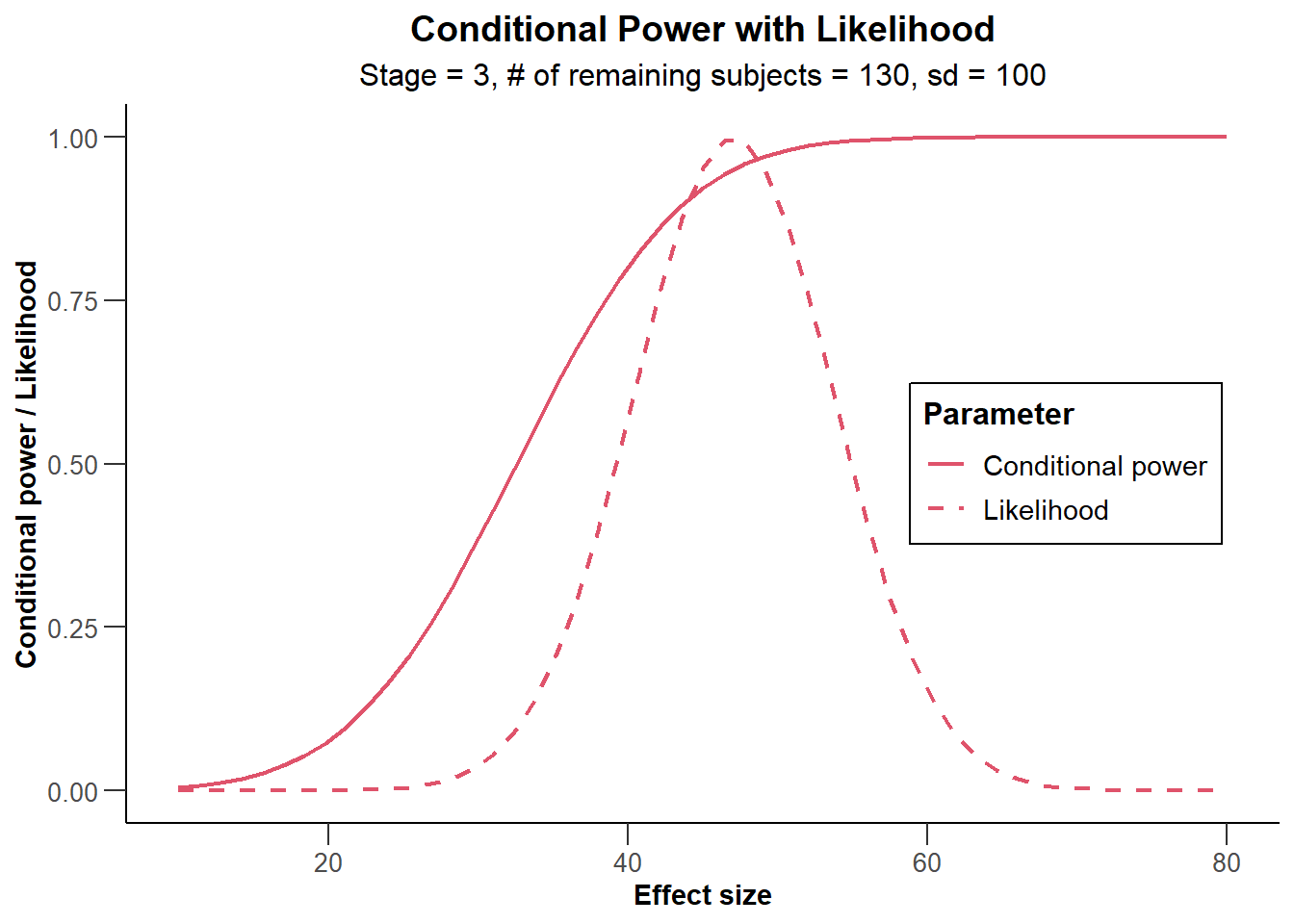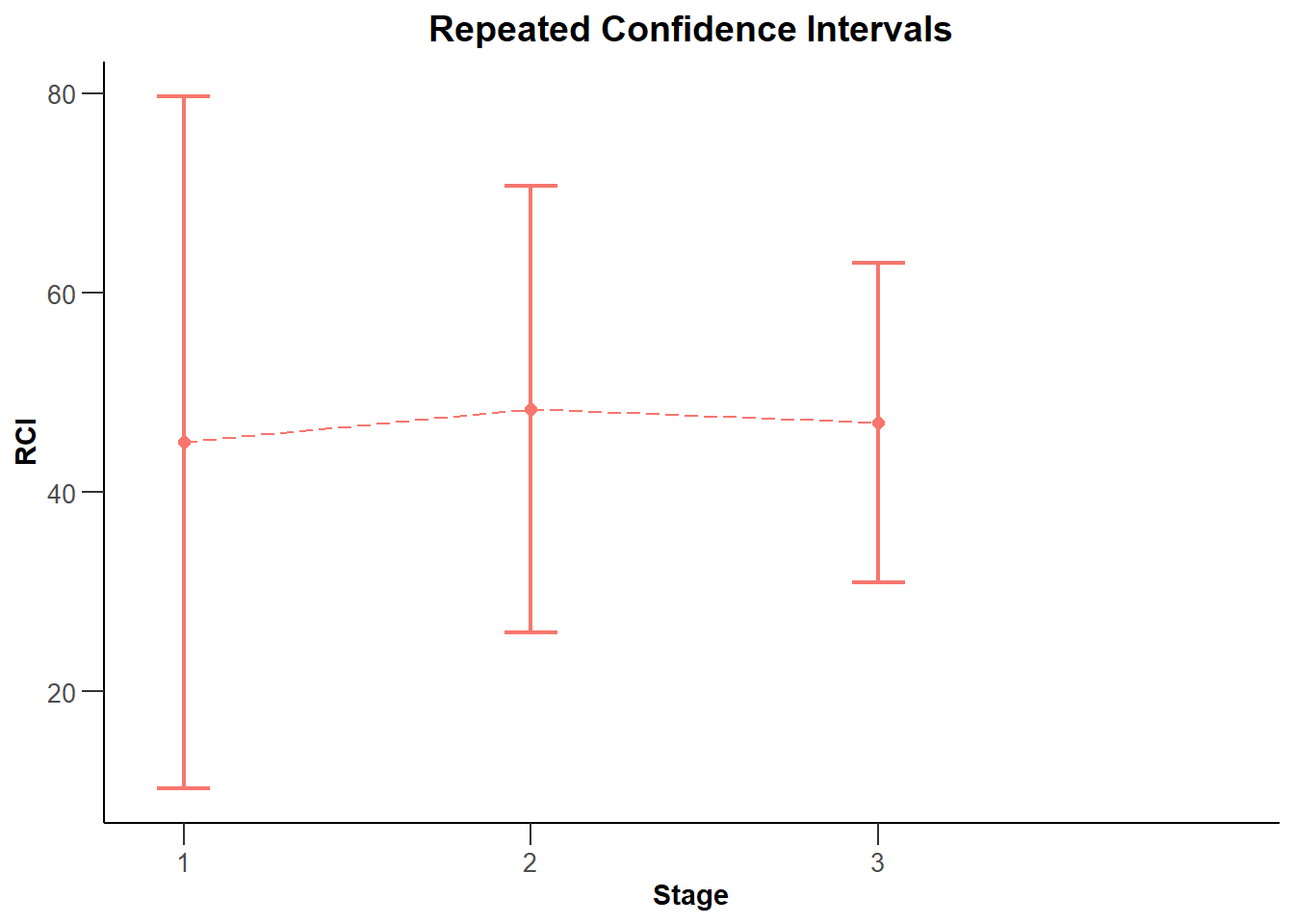Free examples and use-cases:   rpact vignettes

# Summary

This R Markdown document provides many different examples for creating one- and multi-arm analysis result plots with rpact and ggplot2.

# 1 Preparation and design

``````library(rpact)
packageVersion("rpact") ``````
``##  '3.2.1'``

## 1.1 Create a design

``````designIN <- getDesignInverseNormal(kMax = 4, alpha = 0.02,
futilityBounds = c(-0.5, 0, 0.5),   bindingFutility = FALSE,
typeOfDesign = "asKD", gammaA = 1.2,
informationRates = c(0.15, 0.4, 0.7, 1))``````

# 2 Analysis results base

## 2.1 Analysis results base - means

``````simpleDataExampleMeans1 <- getDataset(
n = c(120, 130, 130),
means = c(0.45, 0.51, 0.45) * 100,
stDevs = c(1.3, 1.4, 1.2) * 100
)

x <- getAnalysisResults(design = designIN, dataInput = simpleDataExampleMeans1,
nPlanned = 130, thetaH0 = 30, thetaH1 = 60, assumedStDev = 100)``````
``## Calculation of final confidence interval performed for kMax = 4 (for kMax > 2, it is theoretically shown that it is valid only if no sample size change was performed)``
``plot(x, thetaRange = c(10,80)) ````plot(x, type = 2) ````````simpleDataExampleMeans2 <- getDataset(
n1 = c(23, 13, 22, 13),
n2 = c(22, 11, 22, 11),
means1 = c(2.7, 2.5, 4.5, 2.5) * 100,
means2 = c(1, 1.1, 1.3, 1) * 100,
stds1 = c(1.3, 2.4, 2.2, 1.3) * 100,
stds2 = c(1.2, 2.2, 2.1, 1.3) * 100
)

x <- getAnalysisResults(design = designIN, dataInput = simpleDataExampleMeans2,
thetaH0 = 110, equalVariances = TRUE, directionUpper = TRUE, stage = 2)
plot(x, nPlanned = c(20, 30))``````